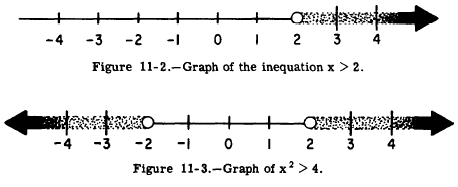Graphing InequalitiesCustom SearchGRAPHING INEQUALITIES An inequation such as x > 2 can be graphed on a number line, as shown in figure 11-2. The heavy line in figure 11-2 contains all values of x which comprise the solution set. Notice that this line continues indefinitely in the positive direction as indicated by the arrow head. Notice also that the point representing x = 2 is designated by a circle. This signifies that the solution set does not contain the number 2. Figure 11-3 is a graph of the inequation x2 > 4. Since the square of any number greater than 2 is greater than 4, the solution set contains all values of x greater than 2. Further less than -2. This is because the square of any negative number smaller than -2 is a positive number greater than 4.Integrated Publishing, Inc. - A (SDVOSB) Service Disabled Veteran Owned Small Business